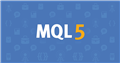# Indicator works but does not update on new bars10

```int OnInit()
{
//--- indicator buffers mapping
SetIndexBuffer(0,SlopeBuffer);
SetIndexBuffer(1,SMABuffer);

//---
return(INIT_SUCCEEDED);
}
//+------------------------------------------------------------------+
//| Custom indicator iteration function                              |
//+------------------------------------------------------------------+
int OnCalculate(const int rates_total,
const int prev_calculated,
const datetime &time[],
const double &open[],
const double &high[],
const double &low[],
const double &close[],
const long &tick_volume[],
const long &volume[],
{
//---
int uncalculatedBar = rates_total - prev_calculated;
for (int i = 0;1<uncalculatedBar;i++)
{
SlopeBuffer[i] = ((iMA(NULL,0,20,0,MODE_SMA,PRICE_CLOSE,i)) - (iMA(NULL,0,20,0,MODE_SMA,PRICE_CLOSE,i+3)))/0.0004;
}

//--- return value of prev_calculated for next call
return(rates_total);
}
```

Hello

I am very new to MT and coding.  I have looked at all the threads on this topic but just dont understand the solutions/fixes.  Can someone pls help.  Thanks in advance.Documentation on MQL5: Constants, Enumerations and Structures / Indicator Constants / Indicators Lines
• www.mql5.com
Some technical indicators have several buffers drawn in the chart. Numbering of indicator buffers starts with 0. When copying indicator values using the CopyBuffer() function into an array of the double type, for some indicators one may indicate the identifier of a copied buffer instead of its number.Moderator
24883

use the code button (Alt+S) when pasting code22754

1. Please edit your (original) post and use the CODE button (Alt-S)! (For large amounts of code, attach it.)
General rules and best pratices of the Forum. - General - MQL5 programming forum 2019.05.06
Messages Editor

2. ```int uncalculatedBar = rates_total - prev_calculated;
for (int i = 0;1<uncalculatedBar;i++)```
After the first run prev_calculated will be equal to rates_total or on a new bar rates_total-1. UncalculatedBar will be at most one, so your loop does nothing. Your look back is 20+3 (iMA)
How to do your lookbacks correctly #9 - #14 & #1910

William Roeder:
1. Please edit your (original) post and use the CODE button (Alt-S)! (For large amounts of code, attach it.)
General rules and best pratices of the Forum. - General - MQL5 programming forum 2019.05.06
Messages Editor

2. After the first run prev_calculated will be equal to rates_total or on a new bar rates_total-1. UncalculatedBar will be at most one, so your loop does nothing. Your look back is 20+3 (iMA)
How to do your lookbacks correctly #9 - #14 & #19

Thanks.  I used the code button as attached the code.

So there seems to be a problem with the loop/loopback.  So what do I need to do to actually fix it?